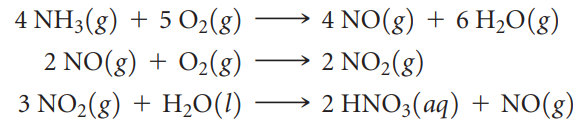×
Get Full Access to Chemistry: The Central Science - 12 Edition - Chapter 4 - Problem 96ae
Get Full Access to Chemistry: The Central Science - 12 Edition - Chapter 4 - Problem 96ae

×

# The commercial production of nitric acid involves theISBN: 9780321696724 27

## Solution for problem 96AE Chapter 4

Chemistry: The Central Science | 12th Edition

• Textbook Solutions
• 2901 Step-by-step solutions solved by professors and subject experts
• Get 24/7 help from StudySoup virtual teaching assistantsChemistry: The Central Science | 12th Edition

4 5 1 279 Reviews
27
0
Problem 96AE

The commercial production of nitric acid involves the following chemical reactions:(a) Which of these reactions are redox reactions? (b) In each redox reaction identify the element undergoing oxidation and the element undergoing reduction.

Step-by-Step Solution:
Step 1 of 3

CEM 141 Lecture 23 – Nuclear Reactions and Bonding Dr. Jian Hu 26 October 2016 Energy changes in nuclear reactions  Nuclear reactions have changes in mass (mass defect), but in chemical reactions, mass is conserved  Mass-energy equivalence E=mc (Einstein) – E represents the “binding energy” that gets released when mass is converted to energy – m represents the mass in kg – c represents the speed of light (3x10 m/s)  Mass defect: mass of the individual nucleons is greater than the mass of the product – mass defect is the difference the mass of the nucleons and the mass of the atom  Mass defect = binding energy  Practice: Each deuterium atom is formed by 1 proton, 1 neutron, and 1 electron. How much energy is released b

Step 2 of 3

Step 3 of 3

##### ISBN: 9780321696724

Unlock Textbook Solution